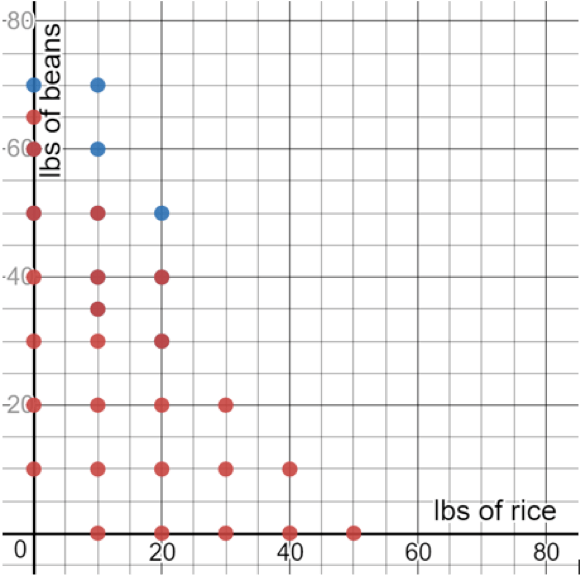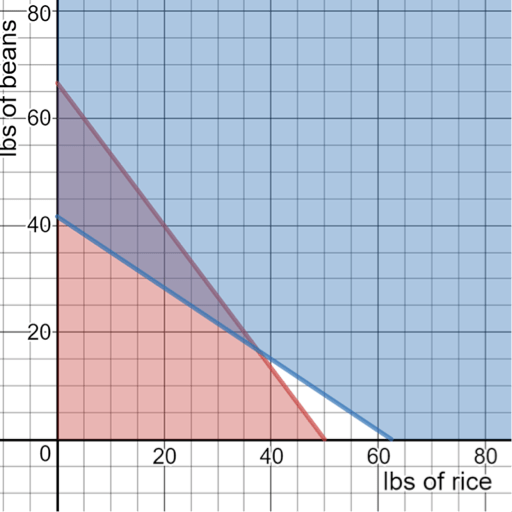# LINR 3 | Lesson 2 | Try This! (Community Event) Solutions

Below shows multiple ways to answer the questions to this problem.

Let $$x=$$ pounds of rice, $$y=$$ pounds of beans.

Table

$$x$$ (lbs rice) $$y$$ (lbs beans) $Servings 0 0 0 ok 0 no 0 10 15 ok 120 no 0 20 30 ok 240 no 0 40 60 ok 480 no 0 50 75 ok 600 ok 0 60 90 720 ok GraphEquations Each lb of rice costs$2, so rice cost is $$2x$$.

Each lb of beans, cost $1.5, so beans cost is $$1.5y$$. Total cost is $$2x+1.5y$$ and we want that to be no more than$100.

$$2x+1.5\leq100$$ dollars

Similarly, we can serve 8 people, for every lb of rice, 8x servings.

And we can serve 12 people for every lb of beans, $$12y$$ servings.

We want to have at least 500 servings or

$$8x+12y\leq500$$ servings

We also can’t have negative pounds or beans or rice so,

$$x\geq0, \, y\geq0$$

Graph using Desmos.com